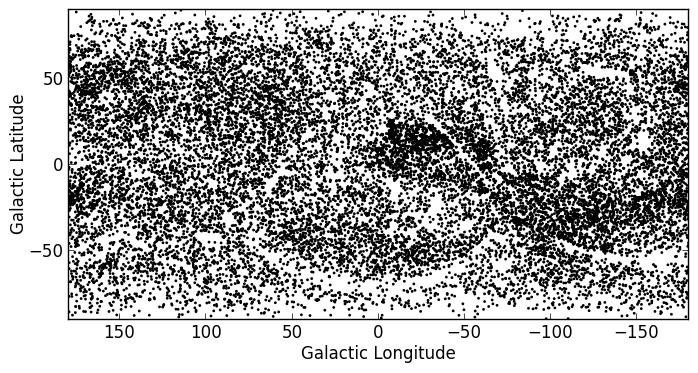Celestial Coordinates¶

Representing and converting coordinates¶

Astropy includes a framework to represent celestial coordinates and transform between them. Astropy most of the common coordinate systems (ICRS, FK4, FK5, and Galactic, AltAz) and users can define their own systems if needed. Transformation of both individual scalar coordinates and arrays of coordinates is supported.

Coordinate objects are instantiated with a flexible and natural approach that supports both numeric angle values and (limited) string parsing:

>>> from astropy.coordinates import SkyCoord
>>> from astropy import units as u
>>> SkyCoord(ra=10.68458, dec=41.26917, unit=(u.degree, u.degree))
<SkyCoord (ICRS): (ra, dec) in deg
(10.68458, 41.26917)>
>>> SkyCoord('00h42m44.3s +41d16m9s')
<SkyCoord (ICRS): (ra, dec) in deg
(10.68458333, 41.26916667)>

The individual components of a coordinate are Angle objects, and their values are accessed using special attributes:

>>> c = SkyCoord(ra=10.68458, dec=41.26917, unit=(u.degree, u.degree))
>>> c.ra
<<Longitude 10.68458 deg>
>>> c.ra.to('hourangle')
<Longitude 0.7123053333333335 hourangle>
>>> c.ra.hms
hms_tuple(h=0.0, m=42.0, s=44.299200000000525)
>>> c.dec
<<Latitude 41.26917 deg>

To convert to some other coordinate system, the easiest method is to use attribute-style access with short names for the built-in systems:

>>> c.galactic
<SkyCoord (Galactic): (l, b) in deg
(121.17424181, -21.57288557)>

The astropy.coordinates subpackage also provides a quick way to get coordinates for named objects (with an internet connection). All coordinate classes have a special class method, from_name(), that accepts a string and queries Sesame to retrieve coordinates for that object:

>>> c_eq = SkyCoord.from_name("M16")
>>> c_eq
<SkyCoord (ICRS): (ra, dec) in deg
(274.7, -13.8067)>

Note

This is intended to be a convenience, and is very simple. If you need precise coordinates for an object you should find the appropriate reference for that measurement and input the coordinates manually.

Practical Exercises¶

Excercise 1

Find the coordinates of the Crab Nebula in ICRS coordinates, and convert them to Galactic Coordinates

Click to Show/Hide Solution

>>> from astropy import coordinates as coord
>>> crab = SkyCoord.from_name('M1')
>>> print(crab)
<SkyCoord (ICRS): (ra, dec) in deg
(83.633083, 22.0145)>
>>> crab_gal = crab.galactic
>>> print(crab_gal)
<SkyCoord (Galactic): (l, b) in deg
(184.55745771, -5.78435696)>

Excercise 2

Using the ROSAT Point source catalog (from excercise 1 in Tabular data), convert all the equatorial coordinates to Galactic coordinates, and make a new plot (but don’t worry about the bright sources).

Click to Show/Hide Solution

from astropy import units as u
from astropy.coordinates import SkyCoord
from astropy.table import QTable
from matplotlib import pyplot as plt

eq = SkyCoord(t['RAJ2000'], t['DEJ2000'])
gal = eq.galactic

fig = plt.figure()